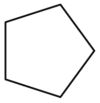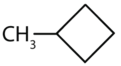# 11.10: Drawing and Naming Cycloalkanes

$$\newcommand{\vecs}{\overset { \rightharpoonup} {\mathbf{#1}} }$$ $$\newcommand{\vecd}{\overset{-\!-\!\rightharpoonup}{\vphantom{a}\smash {#1}}}$$$$\newcommand{\id}{\mathrm{id}}$$ $$\newcommand{\Span}{\mathrm{span}}$$ $$\newcommand{\kernel}{\mathrm{null}\,}$$ $$\newcommand{\range}{\mathrm{range}\,}$$ $$\newcommand{\RealPart}{\mathrm{Re}}$$ $$\newcommand{\ImaginaryPart}{\mathrm{Im}}$$ $$\newcommand{\Argument}{\mathrm{Arg}}$$ $$\newcommand{\norm}{\| #1 \|}$$ $$\newcommand{\inner}{\langle #1, #2 \rangle}$$ $$\newcommand{\Span}{\mathrm{span}}$$ $$\newcommand{\id}{\mathrm{id}}$$ $$\newcommand{\Span}{\mathrm{span}}$$ $$\newcommand{\kernel}{\mathrm{null}\,}$$ $$\newcommand{\range}{\mathrm{range}\,}$$ $$\newcommand{\RealPart}{\mathrm{Re}}$$ $$\newcommand{\ImaginaryPart}{\mathrm{Im}}$$ $$\newcommand{\Argument}{\mathrm{Arg}}$$ $$\newcommand{\norm}{\| #1 \|}$$ $$\newcommand{\inner}{\langle #1, #2 \rangle}$$ $$\newcommand{\Span}{\mathrm{span}}$$

## Learning Objectives

• To name cycloalkanes given their formulas and write formulas for these compounds given their names.

The cycloalkanes—cyclic hydrocarbons with only single bonds—are named by adding the prefix cyclo- to the name of the open-chain compound having the same number of carbon atoms as there are in the ring. Thus the name for the cyclic compound C4H8 is cyclobutane. The carbon atoms in cyclic compounds can be represented by line-angle formulas that result in regular geometric figures. Keep in mind, however, that each corner of the geometric figure represents a carbon atom plus as many hydrogen atoms as needed to give each carbon atom four bonds.Some cyclic compounds have substituent groups attached. Example $$\PageIndex{1}$$ interprets the name of a cycloalkane with a single substituent group.

## Example $$\PageIndex{1}$$

Draw the structure for each compound.

1. cyclopentane
2. methylcyclobutane

### Solution

1. The name cyclopentane indicates a cyclic (cyclo) alkane with five (pent-) carbon atoms. It can be represented as a pentagon.• The name methylcyclobutane indicates a cyclic alkane with four (but-) carbon atoms in the cyclic part. It can be represented as a square with a CH3 group attached.• ## Exercise $$\PageIndex{1}$$

Draw the structure for each compound.

1. cycloheptane
2. ethylcyclohexane

11.10: Drawing and Naming Cycloalkanes is shared under a not declared license and was authored, remixed, and/or curated by LibreTexts.